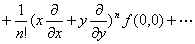2. 多变量函数的泰勒公式

[泰勒公式]  假定在某一点(x0,y0)的邻域D内二元函数f(x,y)有直到n+1阶为止的一切连续偏导数.分别给xy以改变量hk,使连结点(x0,y0)(x0+h,y0+k)的直线段不越出D,那末f (x,y)D内可表成形式:

f (x0+h,y0+k)

`      =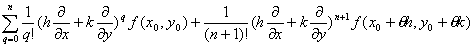`

(0<θ<1)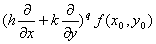==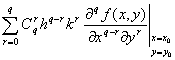20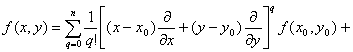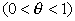特别,x0=0,y0=0,得到

[马克劳林公式]

f (x,y)=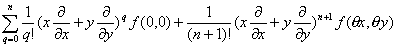[泰勒级数]  在上面泰勒公式,如果把展开式进行到(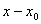)()的任意高的乘幂,则有

f (x,y)=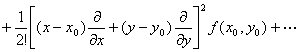[马克劳林级数]  在上面马克劳林公式中,如果把展开式进行到x,y的任意高的乘幂,则有

f (x,y)= f (0,0)+